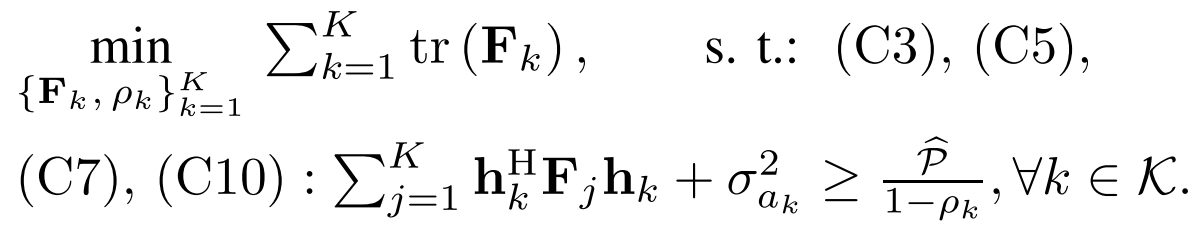# Why do i just mutiply two scalar,but the window show me i need a square ,and error using in .*?

I ran a cvx code,but the window show me there is a error in this code,but i don’t know why

c10_left_hand_up = 0;
sum_10_up = 0;
for j = 1:K
sum_10_up= sum_10_up +  h_k{k}' * Fk_up{j} * h_k{k};
end
c10_left_hand_up = real(c10_left_hand_up + sum_10_up+nois_var_ak_2pow(1))*(1-rho_k_up(k))
c10_right_hand_up=hat_p_up
c10_left_hand_up>=  c10_right_hand_up


Both Fk_up and rho_k_up are variables i define.
Fk_up{j} is the 4 by 4 matrix ,and rho_k_up(k) is a scalar.
Both real(c10_left_hand_up + sum_10_up+nois_var_ak_2pow(1)) and (1-rho_k_up(k)) are scalar

However,the window show me this error

Error using .* (line 262)
Disciplined convex programming error:
Invalid quadratic form(s): not a square.

Error in * (line 36)
z = feval( oper, x, y );

Error in op4_Pp_P_TP (line 89)
c10_left_hand_up =real(c10_left_hand
_up +sum_10_up+nois_var_ak_2pow(1))*(1-rho_k_up(k))

Now i just multiply two scalar,why does the window show me error using .* and it seems that i need a square ? i don’t understand,can anyone help me?

Both Fk_up and rho_k_up are what i want to know,so i can’t put them in the denominator

I commend you to read Why isn't CVX accepting my model? READ THIS FIRST! . , CVX does not allow different variables to be multiplied togetherm except when in gp mode, whicg comes with its own special restrictions.

Have you forgotten Cannot perform the operation: {real affine} .* {convex},but i don't use the .* ? You have violated CVX’s rules, so you get an error message. The error message is not always that informative as to what the actual offending item is.

but this time the error didn’t show meCannot perform the operation: {real affine} .* {convex} ,In C10,i can neither put the 1-rho_k_up(k) in the denominator nor multiply real(c10_left_hand_up + sum_10_up+nois_var_ak_2pow(1)) and (1-rho_k_up(k)) .How can i describe this formula in cvx?

Presuming \rho_k < 1, use
rho_hat*inv_pos(1 - rho_k)
for the RHS of the constraint. I’ll let you fix up the variable names, indexing, etc.

help inv_pos

inv_pos Reciprocal of a positive quantity.
inv_pos(X) returns 1./X if X is positive, and +Inf otherwise.
X must be real.

 For matrices and N-D arrays, the function is applied to each element.

Disciplined convex programming information:
inv_pos is convex and nonincreasing; therefore, when used in CVX
specifications, its argument must be concave (or affine).

but the meaning of using inv_pos (1-rho_k) and 1/(1-rho_k) are the same.the cvx can just accept inv_pos (1-rho_k) only.
as i said,cvx can’t find the variable value if we put this variable into the denominator,so if we replace 1/(1-rho_k) with inv_pos (1-rho_k) ,the rho_k is still in the denominator.

CVX will accept the form I gave. You have to decide if you are o.k. with inv_pos(1-rho_k) being +Inf if rho_k > 1.

If you don’t like CVX’s rules, you are free to create your own tool which is more flexible and will make more transformations automatically for the user.

I know and agree cvx can accept the form you gave,but it can’t optimize and calculate that rho_k value,so replacing 1/(1-rho_k) with inv_pos (1-rho_k) is meaningless for me,there is a constraint that let therho_k <=1 and rho_k >=0,but i did’t show this

I don;t understand why this use of inv_pos is not acceptable to you. You must declare rho_k (or whatever you call it) to be a (CVX) variable. After cvx_end, if the solver finds a solution to your problem,rho_k will have been optimized, and its optimal value will be inserted into rho_k for your use.

After the running of the code,cvx can’t find a value usually,so my teacher told me she remember that if we put a variable into the denominator,the cvx can’t really find the optimal value of that variable.
accept and be calculated the optimal value are two different things,maybe you think they are the same,but they are not in fact.
Again,i agree the cvx can accept the form,inv_pos (1-rho_k),you gave,but it just means the cvx can run your code, it does’t mean cvx can find the rho_k optimal value in this form.

Where else does rho_k appear in your problem?

If your problem is infeasible, that may reflect a deficiency in your modeling or input data, and would not be CVX’s fault.

rho_k appear in c5

that may reflect a deficiency in your modeling or input data,what does this mean?you mean i need more constraint?or i have to let one of rho_k and Fk_up{j} to be non-variable?

I have no idea what your model is supposed to represent. I have no idea what input data values are meaningful or reflective of some real world situation. This forum is not a forum on creating models for electrical engineering, or whatever this is. For that, you should consult subject matter experts, such as perhaps (hopefully) your teacher. Or if you have taken this from a paper, then consider consulting the authors of the paper.

If your problem is infeasible, then one or more constraints are too stringent. Of course, the problem may be feasible for some values of input data and not for others.

Paper link: https://arxiv.org/pdf/1805.08898.pdf ,can you please see the op4 in page 6?you will know what the model i am supposed to represent

I don’t know what any of that represents, or how the P 's are related to the variables, and what that means for how if at all it can be entered into CVX, nor do I care to put in the time and effort to become knowledgeable in this subject area.